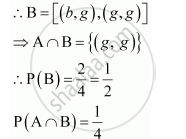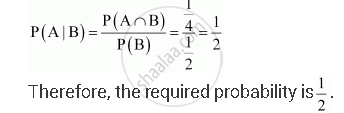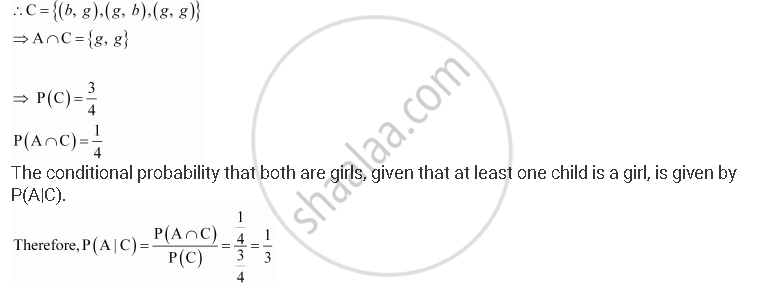# Assume that Each Born Child is Equally Likely to Be a Boy Or a Girl. If a Family Has Two Children, What is the Conditional Probability that Both Are Girls? Give that - Mathematics

Assume that each born child is equally likely to be a boy or a girl. If a family has two children, what is the conditional probability that both are girls? Give that
(i) the youngest is a girl.
(ii) at least one is a girl.

#### Solution 1

Let Bi and Gi, representing the ith child, be a boy and a girl, respectively.
Then, the sample space can be expressed as follows:
S = {B1B2, B1G2, G1B2, G1G2}
Consider the following events:
A = both the children are girls
B = one of the child is a girl
C = the youngest child is a girl
Then, we have:
A = {G1G2}
P(A) = 1/4
B = {B1G2, G1B2, G1G2}
P(B) = 3/4
Also, C = {B1G2, G1G2}
P(C) = 2/4=1/4

So, A∩B = {G1G2} ⇒P(A∩B)=1/4and A∩C = {G1G2} ⇒P(A∩C)=1/4

Now, we have:

(i) Probability that both the children are girls given that the youngest child is a girl:

  P(A/C) = P(A∩C)P(C)=(1/4)/(1/2)=1/2

(ii) Probability that both are girls given that at least one is a girl:

      P(A/B) = P(A∩B)P(B)=(1/4)/(3/4)=1/3

#### Solution 2

Let b and g represent the boy and the girl child respectively. If a family has two children, the sample space will be

S = {(bb), (bg), (gb), (g, g)}

Let A be the event that both children are girls.

:. A = {(g,g)}

(i) Let B be the event that the youngest child is a girl.The conditional probability that both are girls, given that the youngest child is a girl, is given by P (A|B).(ii) Let C be the event that at least one child is a girl.Concept: Conditional Probability
Is there an error in this question or solution?

#### APPEARS IN

NCERT Class 12 Maths
Chapter 13 Probability
Q 12 | Page 539